# SAT II Math I : Types of Numbers

## Example Questions

### Example Question #1 : Types Of Numbers

Express 374 in base six.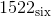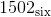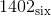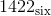Explanation:

To convert a base ten number to base six, divide the number by six, with the remainder being the digit in the units place; continue, dividing each successive quotient by 6 and putting the remainder in the next position to the left until the final quotient is less than 6.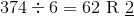- the rightmost digit is 2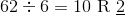- the next digit to the left is 2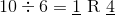- the next digit to the left is 4, and the digit to the left of that is 1.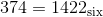### Example Question #2 : Types Of Numbers

Express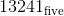in base ten.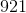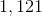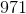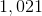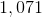Explanation:

Place values in the base five system are powers of five rather than powers of ten.is equal to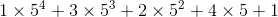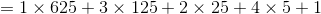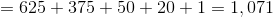### Example Question #3 : Types Of Numbers

Which of the following is the smallest prime number: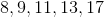?Explanation:

A prime number has only two factor,and itself.

This is not true for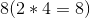or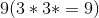so they are not the answer.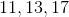are all prime numbers andis the smallest so it is your answer.

### Example Question #1 : Types Of Numbers

Which of the following is a rational number?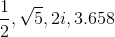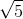None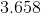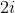A rational number is any number that can be expressed as an integer p or a fraction p/q. In the set of number given onlyfits into that category.